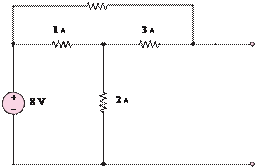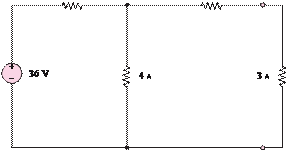### Create an Account

Home / Questions / Find the Thévenin equivalent circuit as seen by the 3 Q resistor for the circuit of Figure...

# Find the Thévenin equivalent circuit as seen by the 3 Q resistor for the circuit of Figure P351 5 Ù 1 Ù 354 Find the Norton equivalent to the left

Find the Thévenin equivalent circuit as seen by the 3-resistor for the circuit of Figure P3.51.

5 Ù                                           1 Ù

3.54  Find the Norton equivalent to the left of  terminals

and of the circuit shown in Figure P3.54.5 Ù

ab

Jun 19 2020 View more View LessSubscribe To Get Solution